# 1 3 2 4 3 2 1 3

• Slides: 16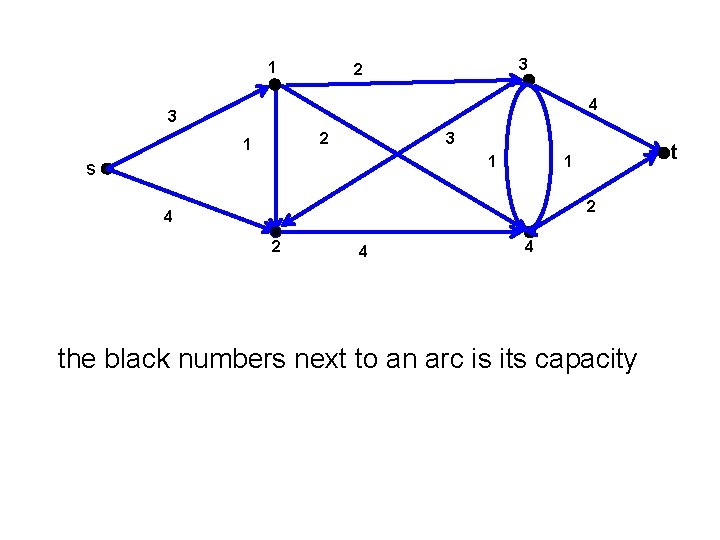1 3 2 4 3 2 1 3 1 s t 1 2 4 4 the black numbers next to an arc is its capacity1 3 1 2 2 4 3 3 2 2 1 3 1 1 s t 1 2 2 1 4 4 the black number next to an arc is its capacity the green number next to an arc is the flow on it extra 3 flow can be sent through (s, 2) and then through (2, 4); all arcs going out of 4 are saturated; but we can reroute 1 flow unit from (1, 4) through (1, 3) and then through (3, t), releasing 1 unit of flow through (4, t) which can then be used for extra flow on the (s, 2)(2, 4)(4, t)-path. To find such possibilities we do the bookkeeping in the so-called residual graph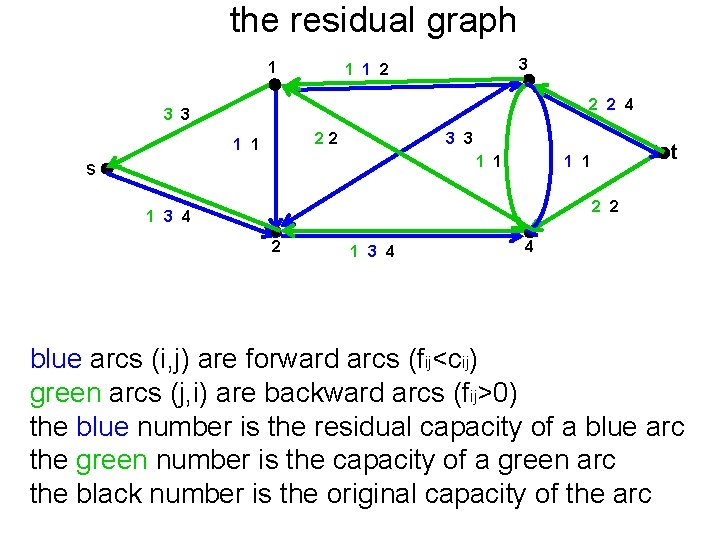the residual graph 1 3 1 1 2 2 2 4 3 3 22 1 1 3 3 1 1 s t 1 1 2 2 1 3 4 4 blue arcs (i, j) are forward arcs (fij<cij) green arcs (j, i) are backward arcs (fij>0) the blue number is the residual capacity of a blue arc the green number is the capacity of a green arc the black number is the original capacity of the arcthe residual graph 1 3 1 1 2 2 3 2 1 3 1 s t 1 2 1 3 red arcs form an augmenting path 4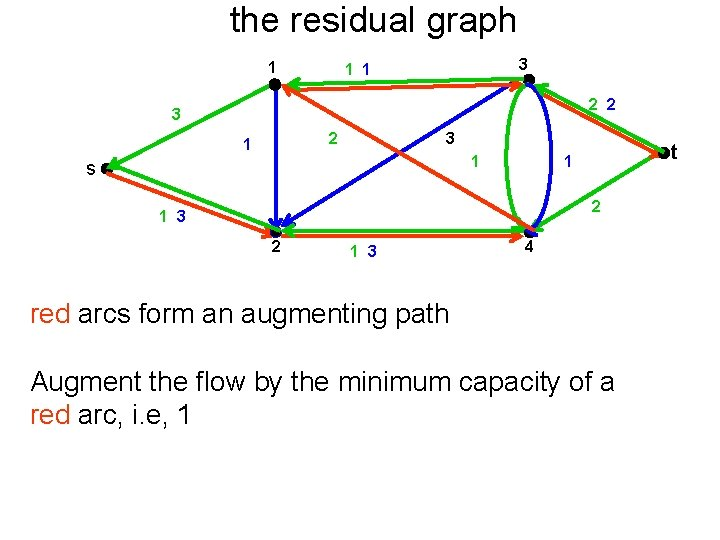the residual graph 1 3 1 1 2 2 3 2 1 3 1 s t 1 2 1 3 4 red arcs form an augmenting path Augment the flow by the minimum capacity of a red arc, i. e, 1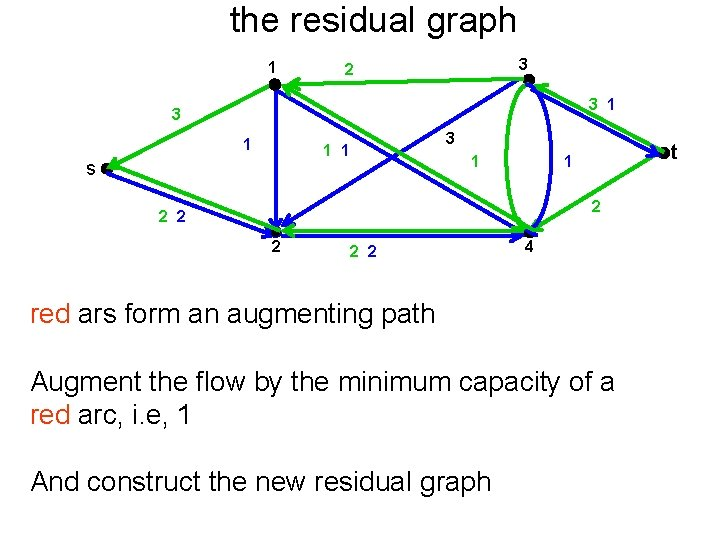the residual graph 1 3 2 3 1 3 1 1 s 1 t 1 2 2 2 4 red ars form an augmenting path Augment the flow by the minimum capacity of a red arc, i. e, 1 And construct the new residual graph1 3 2 4 2 2 1 3 1 s t 1 2 4 4 An s-t cut is defined by a set S of the nodes with s in S and t not in S.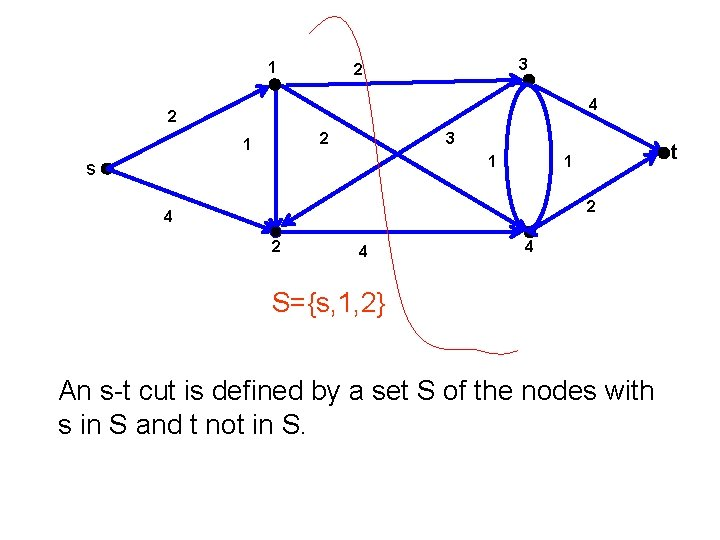1 3 2 4 2 2 1 3 1 s t 1 2 4 4 S={s, 1, 2} An s-t cut is defined by a set S of the nodes with s in S and t not in S.1 3 2 4 2 2 1 3 1 s t 1 2 4 4 S={s, 1, 2} An s-t cut is defined by a set S of the nodes with s in S and t not in S Size of cut S is the sum of the capacities on the arcs from S to NS.1 3 2 4 2 2 1 3 1 s t 1 2 4 4 C(S)=c 13+c 14+c 24= 2+2+4=8 An s-t cut is defined by a set S of the nodes with s in S and t not in S Size of cut S is the sum of the capacities on the arcs from S to NS.S 1 1 3 2 4 2 2 1 3 1 s t 1 2 4 2 C(S 1)=cs 1+c 24= 2+4=6 4 4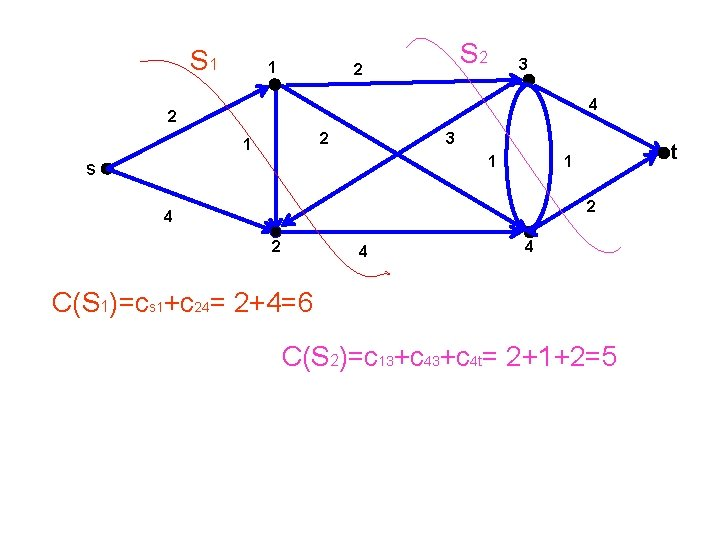S 1 1 S 2 2 3 4 2 2 1 3 1 s t 1 2 4 4 C(S 1)=cs 1+c 24= 2+4=6 C(S 2)=c 13+c 4 t= 2+1+2=5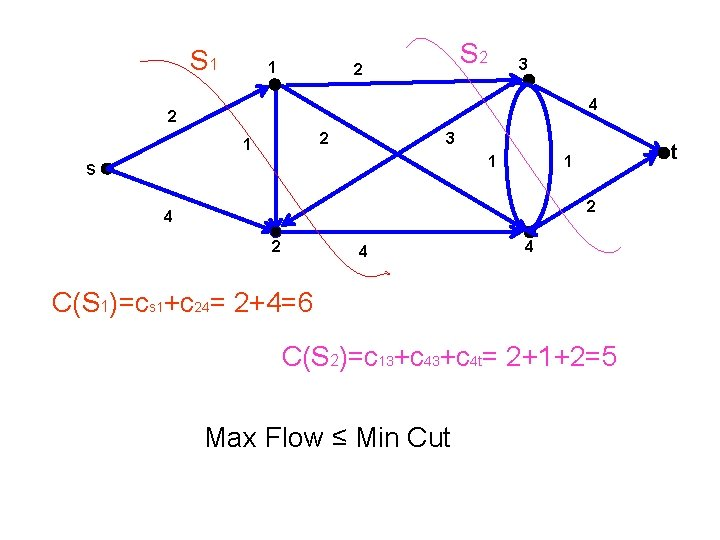S 1 1 S 2 2 3 4 2 2 1 3 1 s t 1 2 4 4 C(S 1)=cs 1+c 24= 2+4=6 C(S 2)=c 13+c 4 t= 2+1+2=5 Max Flow ≤ Min CutS 1 1 S 2 2 3 4 2 2 1 3 1 s t 1 2 4 4 C(S 1)=cs 1+c 24= 2+4=6 C(S 2)=c 13+c 4 t= 2+1+2=5 Max Flow ≤ Min Cut fs 1+fs 2 ≤ Min Cut ≤ 5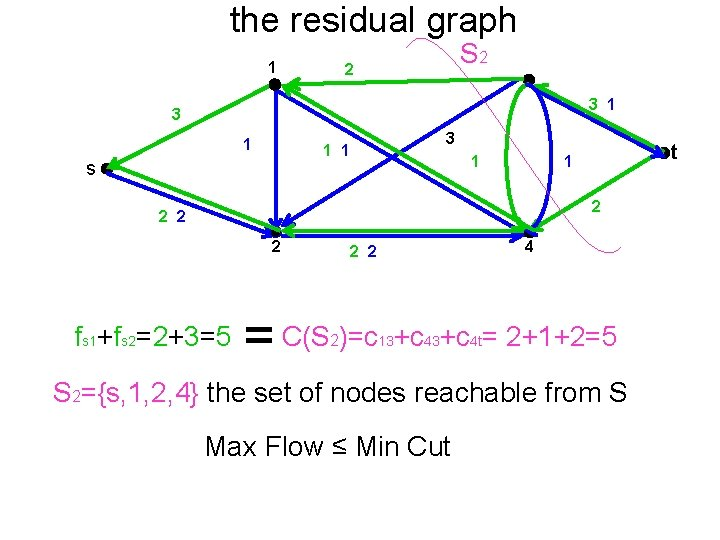the residual graph 1 S 2 2 3 1 3 1 1 s 1 t 1 2 2 fs 1+fs 2=2+3=5 4 2 2 = C(S )=c +c +c = 2+1+2=5 2 13 43 4 t S 2={s, 1, 2, 4} the set of nodes reachable from S Max Flow ≤ Min Cutthe residual graph 1 S 2 2 3 1 3 1 1 s 1 t 1 2 2 fs 1+fs 2=2+3=5 4 2 2 = C(S )=c +c +c = 2+1+2=5 2 13 43 4 t S 2={s, 1, 2, 4} the set of nodes reachable from S Max Flow ≤ Min Cut Theorem: Max Flow = Min Cut